This document contains R versions of the boxed examples from Chapter 7 of the “Analysis and Interpretation of Freshwater Fisheries Data” book. Some sections build on descriptions from previous sections, so each section may not stand completely on its own. More thorough discussions of the following items are available in linked vignettes:

The following additional packages are required to complete all of the examples (with the required functions noted as a comment and also noted in the specific examples below).

> library(FSA)          # Summarize, fitPlot, residPlot
> library(car)          # Anova, recode, leveneTest
> library(Hmisc)        # rcorr
> library(multcomp)     # glht, mcp

In addition, external tab-delimited text files are used to hold the data required for each example. These data are loaded into R in each example with read.table(). Before using read.table() the working directory of R must be set to where these files are located on your computer. The working directory for all data files on my computer is set with

> setwd("C:/aaaWork/Web/fishR/BookVignettes/aiffd2007")

In addition, I prefer to not show significance stars for hypothesis test output, reduce the margins on plots, alter the axis label positions, and reduce the axis tick length. In addition, contrasts are set in such a manner as to force R output to match SAS output for linear model summaries. All of these options are set with

> options(width=90,continue=" ",show.signif.stars=FALSE,
contrasts=c("contr.sum","contr.poly"))
> par(mar=c(3.5,3.5,1,1),mgp=c(2.1,0.4,0),tcl=-0.2)

## 7.1 Detection of Changes in Relative Abundance with Highly Variable Catch per Unit Effort ($$\frac{C}{f}$$) Data

A time series data set was produced to illustrate the effects of highly variable catch-per-unit-effort (CPE; $$\frac{C}{f}$$) data on the ability to detect relative abundance changes for a hypothetical fish population that is declining through time. The first column was year, the second column was population abundance (N) showing a decline of 5% each year, the third column was $$\frac{C}{f}$$ as $$0.001N$$, the fourth column was $$\frac{C}{f}$$ varying randomly by 5% or 10% above and below $$0.001N$$, and the final column was $$\frac{C}{f}$$ varying randomly by 20% or 40% above or below $$0.0001N$$. R code producing the same results as this time series data set is shown below.

### 7.1.1 Setting the Base Abundance and CPE Data

In this example, the population abundance data (column 2) is created by starting with an initial abundance of 10000 individuals and using a 95% annual survival rate. These data are created in R by first creating a vector containing the years (note I will label the years from 0-14 rather than 1-15 for ease) and objects for the initial population size and the annual survival rate. The abundance for each year is then simulate with an exponential decay model and rounded to the nearest whole individual (using round() with a second argument of 0 meaning to “no” decimal places.). The base CPE data in the box was created by dividing the abundance data by 1000.

> year <- 0:14                   # years for simulation
> N0 <- 10000                    # initial population size
> A <- 0.95                      # annual survival rate
> abund <- round(N0*A^year,0)    # abundance from exponential decay model
> cpe <- round(abund/1000,1)     # simulated cpe data
> cbind(year,abund)              # for display only
      year abund
[1,]    0 10000
[2,]    1  9500
[3,]    2  9025
[4,]    3  8574
[5,]    4  8145
[6,]    5  7738
[7,]    6  7351
[8,]    7  6983
[9,]    8  6634
[10,]    9  6302
[11,]   10  5987
[12,]   11  5688
[13,]   12  5404
[14,]   13  5133
[15,]   14  4877

### 7.1.2 Creating the Random CPE Data

The first column of random CPE data is then created by randomly applying a 5 or 10% positive or negative “measurement error” to the base CPE data. These data were created in R by first creating a vector that contained the 5 or 10% positive or negative measurement errors and then randomly selecting (using sample() with the first argument being the vector to randomly sample from, the second argument the number of times to sample, and replace=TRUE indicating to sample with replacement) from this vector as many times as the length of the vector containing the base CPE values. The randomly selected percent measurement errors were then multiplied by the base CPE and added to the base CPE to create the “CPE data with measurement error.

> me1 <- c(-0.10,-0.05,0.05,0.10)
> me1a <- sample(me1,length(cpe),replace=TRUE)
> cpe.me1 <- round(cpe+cpe*me1a,1)

The above code was slightly modified to create the 20 or 40% measurement error data.

> me2 <- c(-0.4,-0.2,0.1,0.4)
> me2a <- sample(me2,length(cpe),replace=TRUE)
> cpe.me2 <- round(cpe+cpe*me2a,1)
> ( d1 <- data.frame(year,abund,cpe,cpe.me1,cpe.me2) )     # for storage
   year abund  cpe cpe.me1 cpe.me2
1     0 10000 10.0     9.5    14.0
2     1  9500  9.5     8.6    10.4
3     2  9025  9.0     8.1    12.6
4     3  8574  8.6     9.0     5.2
5     4  8145  8.1     8.9     4.9
6     5  7738  7.7     6.9     6.2
7     6  7351  7.4     8.1     8.1
8     7  6983  7.0     6.3     9.8
9     8  6634  6.6     7.3     5.3
10    9  6302  6.3     6.9     8.8
11   10  5987  6.0     6.6     3.6
12   11  5688  5.7     5.4     8.0
13   12  5404  5.4     5.1     3.2
14   13  5133  5.1     4.6     4.1
15   14  4877  4.9     4.7     5.4

Finally, a plot of these data more clearly makes the author’s point about variability in CPE data.

> plot(cpe~year,data=d1,ylim=c(0,15),xlab="Year",ylab="CPE",type="l",lwd=2,col="black")
> points(d1$year,d1$cpe.me1,type="b",pch=19,lwd=1,col="blue")
> points(d1$year,d1$cpe.me2,type="b",pch=19,lwd=1,col="red")
> legend("topright",legend=c("Truth","5 or 10%","20 or 40%"),col=c("black","blue","red"),
lwd=c(2,1,1),pch=c(NA,19,19))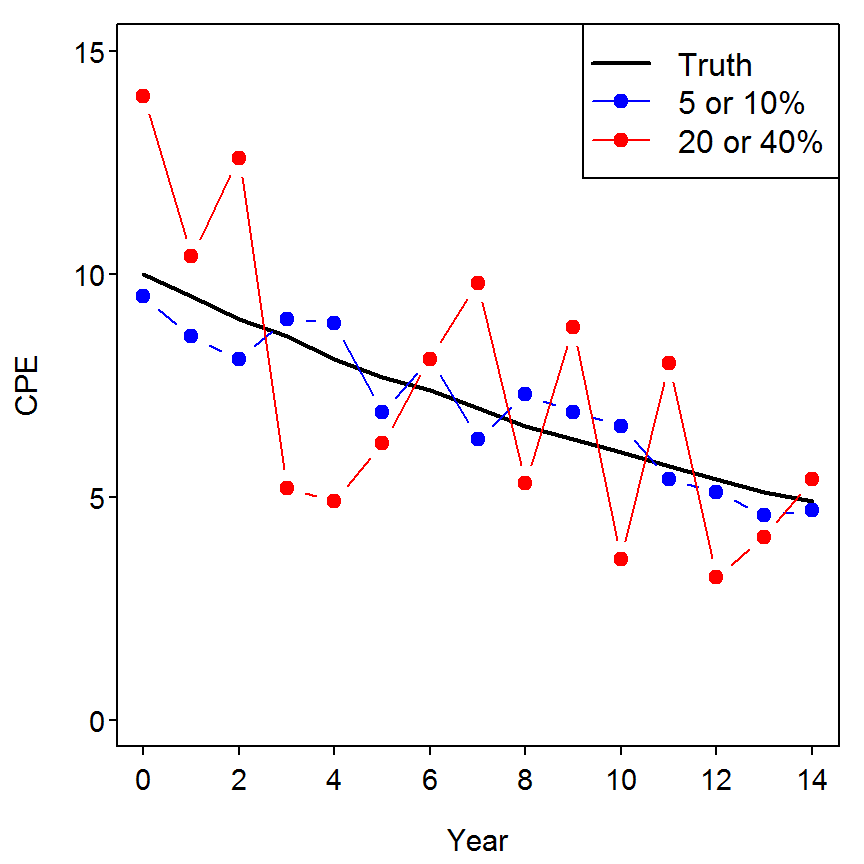### 7.1.3 Try Some Simulations

The following function can be used to more quickly create the plot above and will facilitate simulations.

> cpe.sim <- function(year,cpe,me1,me2=NULL) {
cpe1 <- cpe + cpe*sample(me1,length(cpe),replace=TRUE)
if (!is.null(me2)) cpe2 <- cpe + cpe*sample(me2,length(cpe),replace=TRUE)
max.y <- max(c(cpe,cpe1,cpe2))
plot(year,cpe,ylim=c(0,max.y),xlab="Year",ylab="CPE",type="l",lwd=2,col="black")
points(year,cpe1,type="b",pch=19,lwd=1,col="blue")
if (!is.null(me2)) points(year,cpe2,type="b",pch=19,lwd=1,col="red")
if (is.null(me2)) legend("topright",legend=c("Truth","CPE1"),col=c("black","blue"),lwd=c(2,1),pch=19)
else legend("topright",legend=c("Truth","CPE1","CPE2"),col=c("black","blue","red"),lwd=c(2,1,1),pch=19)
}

A plot similar (different because of the randomization) to the one above can then be created with the following code. If you repeat the code below several times you can see how different randomizations look (and ultimately see that the author’s point did not depend on “their” randomization).

> cpe.sim(d1$year,d1$cpe,me1,me2)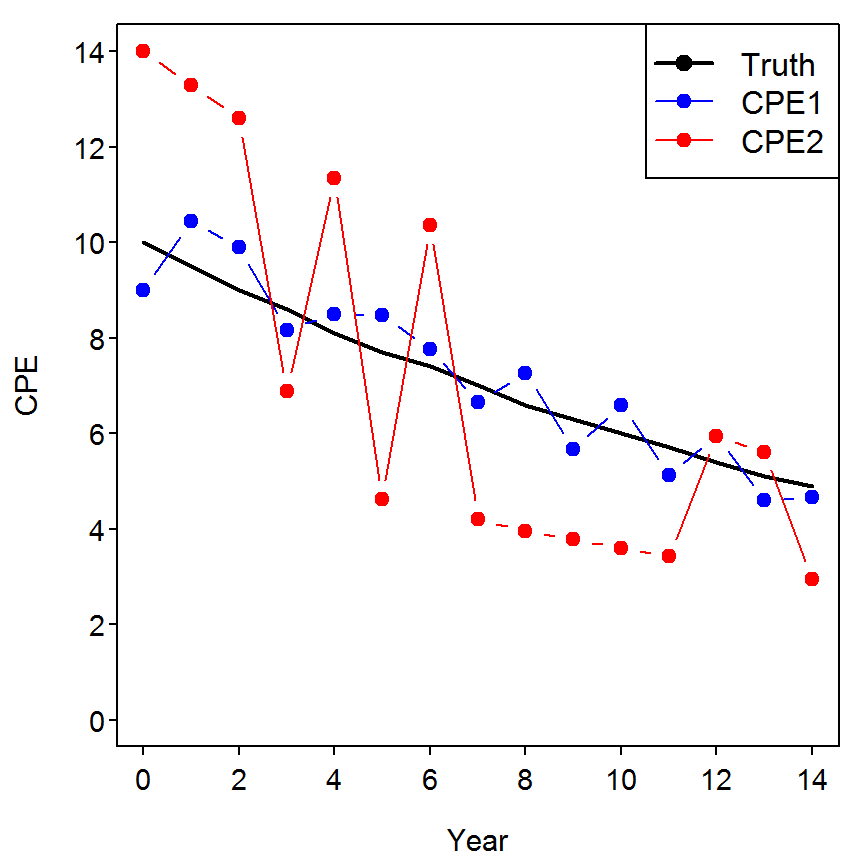## 7.2 Power Analysis Assessment of Sampling Effort

Preliminary sampling of Channel Catfish (Ictalurus punctatus) in two hypothetical small impoundments is conducted with traps in early summer to obtain $$\frac{C}{f}$$ data as the first step in establishing an annual monitoring program to assess temporal variation in mean $$\frac{C}{f}$$. In each reservoir, 20 traps are set at randomly selected locations, left overnight, and retrieved the following day. The following $$\frac{C}{f}$$ data (i.e., fish/trap-night) and statistics are obtained for each impoundment. Each $$\frac{C}{f}$$ value is transformed as $$log_{10}\frac{C}{f}+1$$ to assess the effects of data transformation on $$\frac{C}{f}$$ statistics and estimates of needed sampling effort.

### 7.2.1 Preparing and Summarizing Data

The Box7_2.txt data file is read and observed below. The common logarithms (using log10) of both CPE variables (as described in the text) are also appended to the data frame.

> d2 <- read.table("data/Box7_2.txt",header=TRUE)
> str(d2)
'data.frame':   20 obs. of  3 variables:
$set : int 1 2 3 4 5 6 7 8 9 10 ...$ cpe.A: int  0 0 0 0 0 0 0 0 1 1 ...
$cpe.B: int 1 1 2 2 3 3 3 4 4 4 ... > d2$logcpe.A <- log10(d2$cpe.A+1) > d2$logcpe.B <- log10(d2$cpe.B+1) > str(d2) 'data.frame': 20 obs. of 5 variables:$ set     : int  1 2 3 4 5 6 7 8 9 10 ...
$cpe.A : int 0 0 0 0 0 0 0 0 1 1 ...$ cpe.B   : int  1 1 2 2 3 3 3 4 4 4 ...
$logcpe.A: num 0 0 0 0 0 ...$ logcpe.B: num  0.301 0.301 0.477 0.477 0.602 ...

The authors comment about summary statistics and histograms for both the raw and logged CPE for both collections. These summaries and graphs can be constructed with Summarize() from the FSA package. and hist(). Note that lapply() is used to “apply” Summarize() to each element of a list. The as.list() function is used to convert the data frame (except for the first column) to a list for use lapply().

> lapply(as.list(d2[,-1]),Summarize,digits=3)
$cpe.A n mean sd min Q1 median Q3 max percZero 20.00 3.10 5.19 0.00 0.00 1.00 3.00 20.00 40.00$cpe.B
n     mean       sd      min       Q1   median       Q3      max percZero
20.00     5.40     4.43     1.00     3.00     4.00     6.00    20.00     0.00

$logcpe.A n mean sd min Q1 median Q3 max percZero 20.000 0.385 0.422 0.000 0.000 0.301 0.602 1.322 40.000$logcpe.B
n     mean       sd      min       Q1   median       Q3      max percZero
20.000    0.731    0.254    0.301    0.602    0.699    0.845    1.322    0.000 
> hist(d2$cpe.A,main="")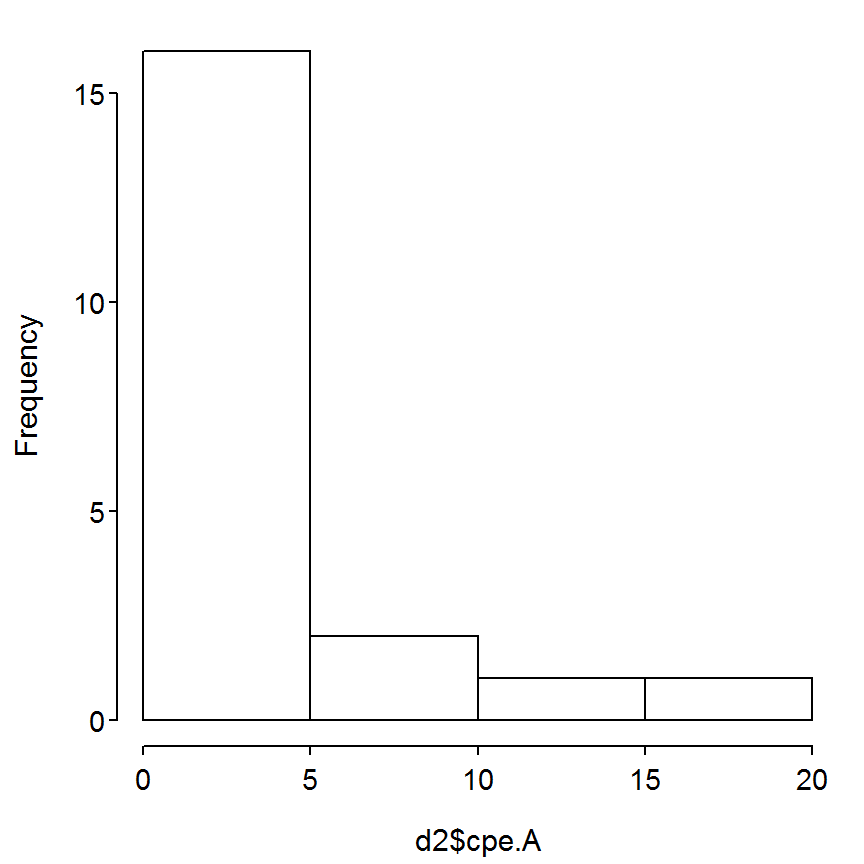> hist(d2$cpe.B,main="")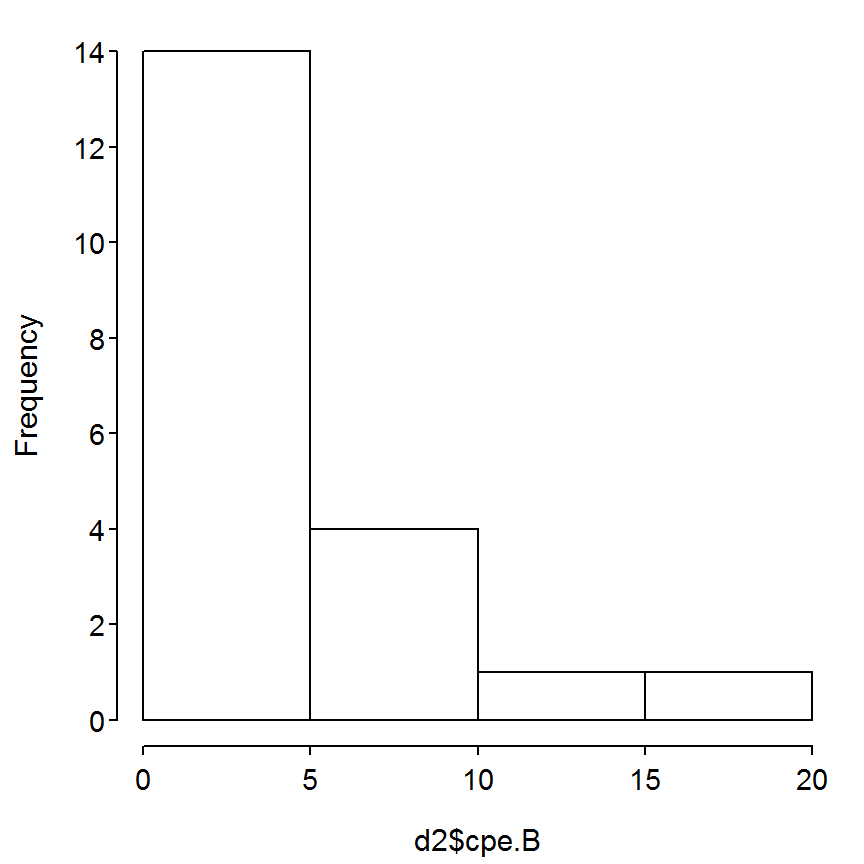> hist(d2$logcpe.A,main="")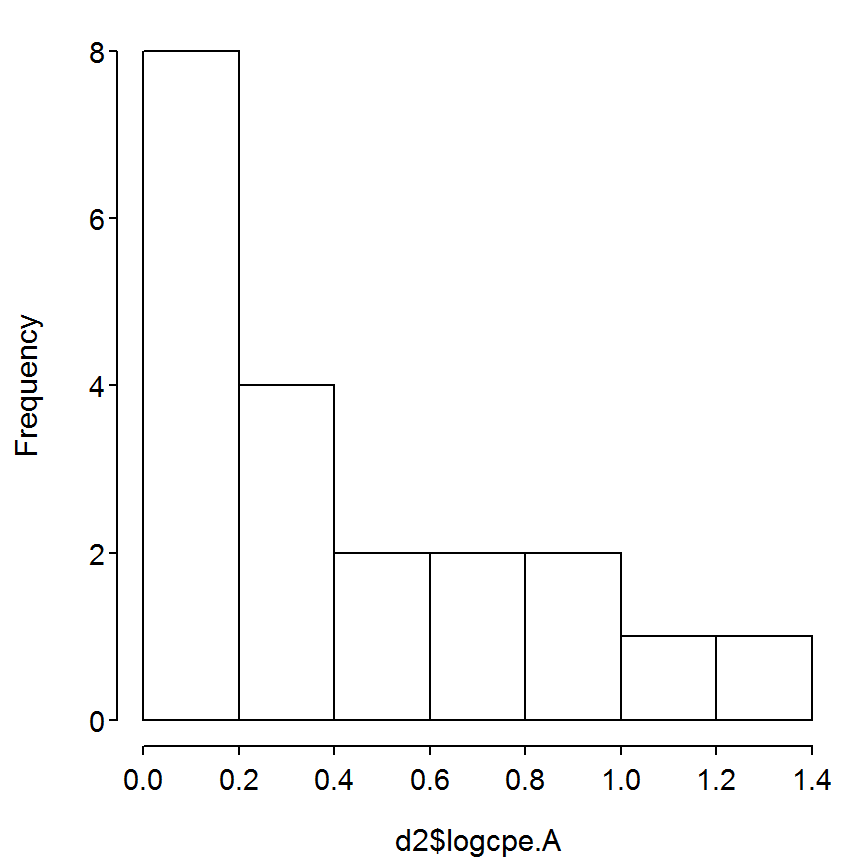> hist(d2$logcpe.B,main="")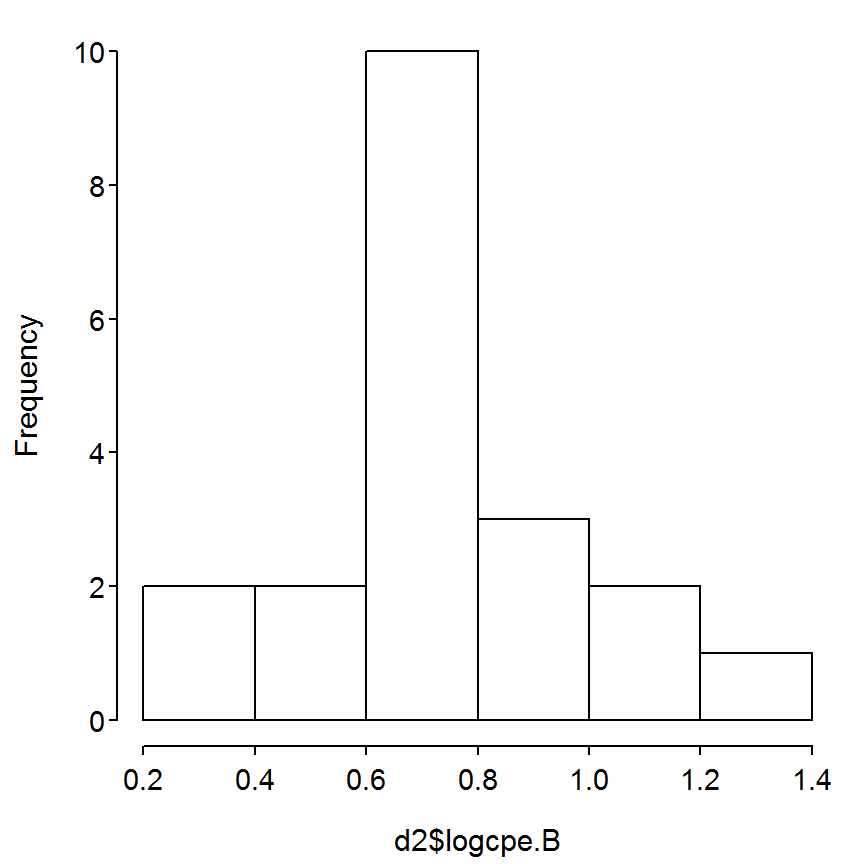### 7.2.2 Power Calculations

The power calculations computed further below will be aided by saving the mean and standard deviation of logged CPE for fish from impoundment impoundment B into objects.

> ( mn.B <- mean(d2$logcpe.B) )  0.7307405 > ( sd.B <- sd(d2$logcpe.B) )
 0.2541973

The sample size required to detect a 10% change in the mean log CPE can be computed with power.t.test(). This function requires a number of arguments as described below.

• delta.mn: The absolute value change in mean to be detected.
• power: The desired level of power ($$1-\beta$$), as a proportion.
• sig.level: The significance level (i.e., $$\alpha$$) to be used.
• sd: The expected standard deviation for the variable.
• type: The type of t-test to be used (i.e. "two.sample" for when comparing means from two samples (e.g., comparing means between two years)).
• alt: The type of alternative hypothesis being examined ("one.sided" corresponds to either the “significant decline” or “significant increase” hypotheses).

The code to determine the sample sizes in the two examples in the box is shown below.

> power.t.test(n=NULL,delta=mn.B*0.10,power=0.9,sig.level=0.05,sd=sd.B,type="two.sample",
alt="one.sided")

Two-sample t test power calculation

n = 207.9394
delta = 0.07307405
sd = 0.2541973
sig.level = 0.05
power = 0.9
alternative = one.sided

NOTE: n is number in *each* group
> power.t.test(n=NULL,delta=mn.B*0.20,power=0.8,sig.level=0.10,sd=sd.B,type="two.sample",
alt="one.sided")

Two-sample t test power calculation

n = 27.70165
delta = 0.1461481
sd = 0.2541973
sig.level = 0.1
power = 0.8
alternative = one.sided

NOTE: n is number in *each* group

The following code is an example illustrating the effect of changing the proportion of population decline to be detected on the required sample size.

> perc.delta <- seq(0.05,0.25,0.01)
> deltas <- mn.B*perc.delta
> p1 <- sapply(deltas,power.t.test,n=NULL,power=0.9,sig.level=0.05,sd=sd.B,type="two.sample",
alt="one.sided")
> p1.n <- as.numeric(p1["n",])
> plot(p1.n~perc.delta,xlab="Proportion Population Decline",ylab="Sample Size",type="l",
ylim=c(0,max(p1.n)))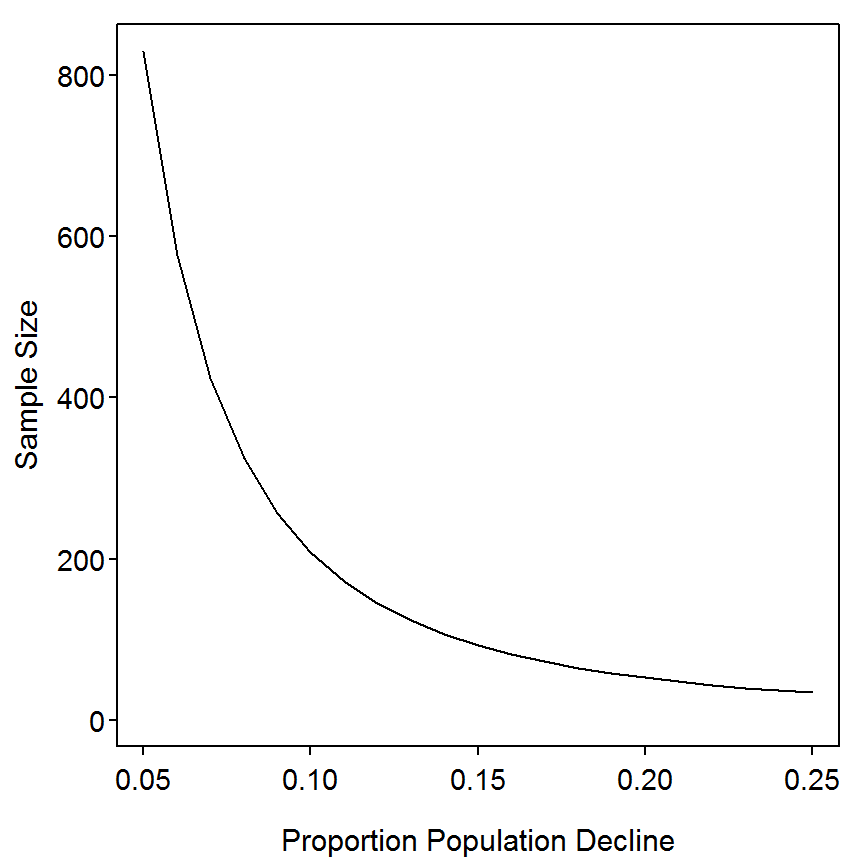## 7.3 Illustraton of Blocked Design in One-Way Analysis of Variance (ANOVA)

An investigator wishes to determine if the abundance of bluegill (Lepomis macrochirus) differs among vegetated and non-vegetated areas of lakes. Bluegills are sampled using trap nets set within a vegetated and a non-vegetated area in each of eight lakes. In this study, the vegetation (presence or absence) is a fixed effect, the blocking factor is lake, and the response is the $$\frac{C}{f}$$ of bluegill (fish/trap-night). Using the following hypothetical data set, we show how ignoring the blocking factor (lake) can lead to erroneous conclusions about the effect of vegetation on the relative abundance of bluegill.

### 7.3.1 Preparing Data

The data for this box was supplied as an Excel file. For simplicity, I rearranged the data in that file and saved the Excel worksheet as a tab-delimited text file to be read into R. The Box7_3.txt data file is read and observed below. To perform an analysis of variance in R the classification variables need to be considered factor variables. The structure of the data frame above indicates that the veg and lake variables are considered to be factors. Thus, no modification of these variables is needed (see Box 3.10 in the Chapter 3 vignette as an example of when a variable must be coerced to be a factor).

> d3 <- read.table("data/Box7_3.txt",header=TRUE)
> str(d3)
'data.frame':   16 obs. of  3 variables:
$veg : Factor w/ 2 levels "absent","present": 1 1 1 1 1 1 1 1 2 2 ...$ lake: Factor w/ 8 levels "A","B","C","D",..: 1 2 3 4 5 6 7 8 1 2 ...
$cpue: int 63 45 30 50 80 67 48 55 70 56 ... ### 7.3.2 Fitting The Model The blocked ANOVA model is fit in R with lm() with a formula of the form response~block+explanatory. The ANOVA table is extracted by submitting the saved lm object to anova(). A visual representation of the model is constructed with fitPlot() from the FSA package. which requires the saved lm object as its first argument (The interval=FALSE argument was used because the sample size is not large enough to compute proper CIs for each group. In addition, the x-axis can be labeled as usual and the default main graphic label can be suppressed by including main="".). > lm1 <- lm(cpue~lake+veg,data=d3) > anova(lm1)  Df Sum Sq Mean Sq F value Pr(>F) lake 7 3023.9 431.99 35.394 6.069e-05 veg 1 315.1 315.06 25.814 0.00143 Residuals 7 85.4 12.21 Total 15 3424.4  > fitPlot(lm1,xlab="Lake",main="",interval=FALSE)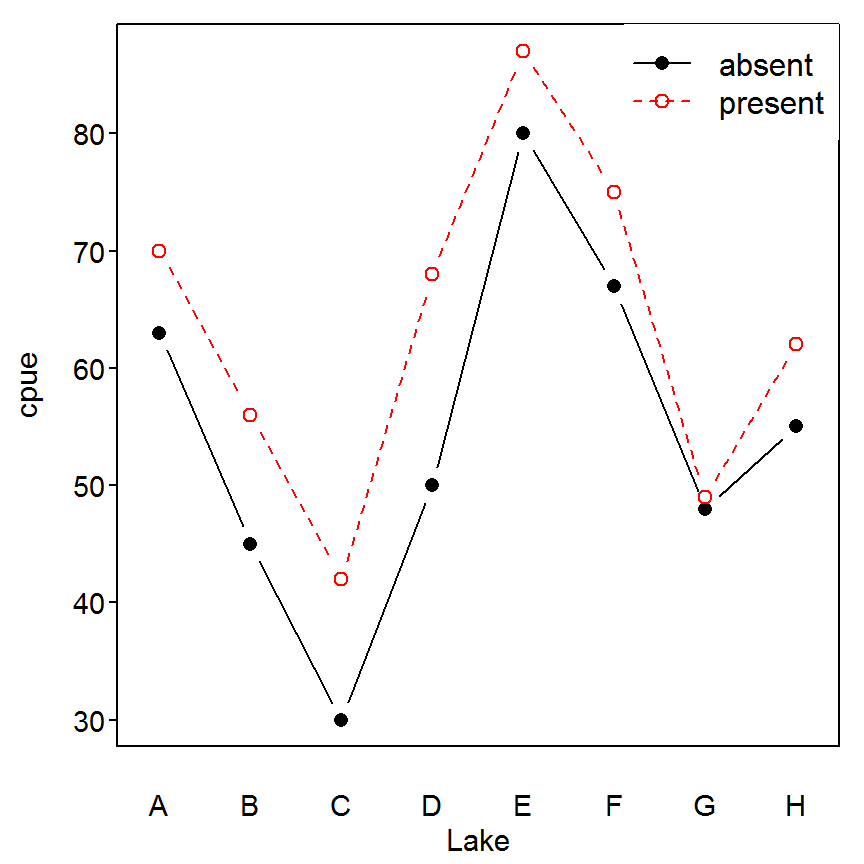### 7.3.3 Non-Blocked ANOVA model The effect of ignoring the block (i.e., lake) in the ANOVA model was illustrated in the box. This model is fit in R and summarized as shown below. > lm2 <- lm(cpue~veg,data=d3) > anova(lm2)  Df Sum Sq Mean Sq F value Pr(>F) veg 1 315.1 315.06 1.4186 0.2534 Residuals 14 3109.4 222.10 Total 15 3424.4  > fitPlot(lm2,xlab="Vegetation Treatment",main="")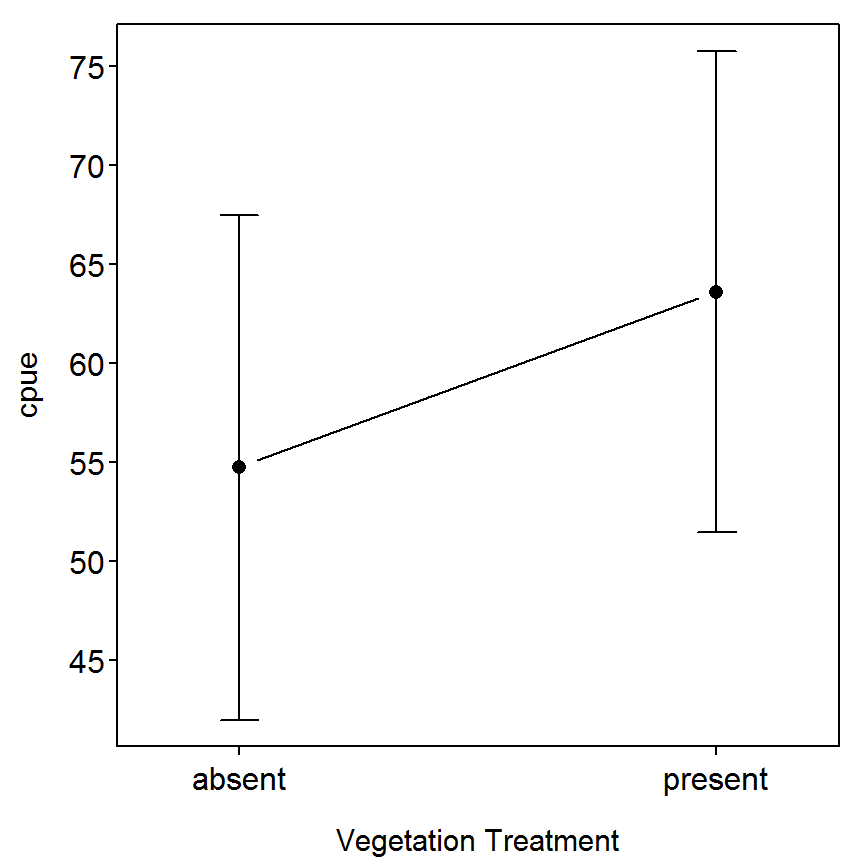## 7.4 Analysis of $$\frac{C}{f}$$ Data from a Temporal Monitoring Program Not Yet Converted ## 7.5 Assessment of Depth Distribution Patterns of Yellow Perch based on $$\frac{C}{f}$$ Data Data on $$\frac{C}{f}$$ (fish/net/h) of Yellow Perch (Perca flavescens) captured with gill nets in a Midwestern lake were obtained during midday at five depths during two months with three randomly selected sites sampled at each depth during each month. A two-way ANOVA was used to assess effects of sampling depth and month as well as the interaction between the two. ### 7.5.1 Preparing Data The Box7_5.txt data file is read and observed below. The structure of the data frame indicates that the Month and Depth variables are not considered to be factor variables (or class variables in SAS). It is important that these variables are factors rather than integers so that lm() in R will perform a two-way ANOVA rather than a multiple linear regression. A variable is converted to a factor with factor(). Additionally, I would prefer that the Month variable is shown as “named” months rather than numbers (the text shows that 1=June and 2=August). The recode() function is from the car package. Also note that Hmisc has a recode() function. If Hmisc is loaded after car then the Hmisc version will be used. This can be prevented by loading car after Hmisc or explicitly telling R to use the car version with car::recode(). provides a methodology for creating a new variable that is a recoding of an old variable. This function requires the original variable as it’s first argument and a string indicating how to recode that variable as its second argument(This function is very flexible and you should consult its help page for a more thorough description.). R defaults to order the levels of a factor in alphabetical order. The order can be manually set with the levels= argument as illustrated below. > d5 <- read.table("data/Box7_5.txt",header=TRUE) > str(d5) 'data.frame': 30 obs. of 3 variables:$ Month: int  1 1 1 1 1 1 1 1 1 1 ...
$Depth: num 2.5 2.5 2.5 5 5 5 10 10 10 15 ...$ CPE  : int  2 4 7 6 10 12 8 12 33 10 ...
> d5$fDepth <- factor(d5$Depth)
> d5$fMonth <- car::recode(d5$Month,"1='June'; 2='August'",as.factor.result=TRUE)
> d5$fMonth <- factor(d5$fMonth,levels=c("June","August"))
> str(d5)
'data.frame':   30 obs. of  5 variables:
$Month : int 1 1 1 1 1 1 1 1 1 1 ...$ Depth : num  2.5 2.5 2.5 5 5 5 10 10 10 15 ...
$CPE : int 2 4 7 6 10 12 8 12 33 10 ...$ fDepth: Factor w/ 5 levels "2.5","5","10",..: 1 1 1 2 2 2 3 3 3 4 ...
$xvals[CI.seln], : zero-length arrow is of indeterminate angle and so skipped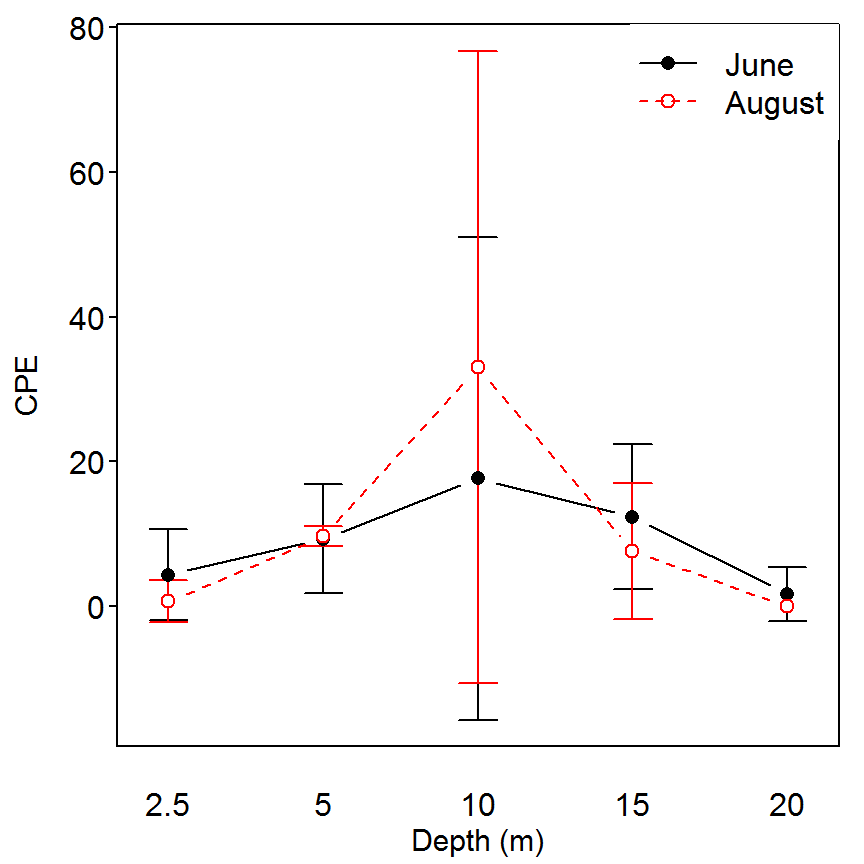The results above suggested that the interaction and month main effects were not significant but that the depth main effect was significant. This result suggests that there is some difference in mean CPE among the five depths. Tukey’s multiple comparison procedure, implemented through glht() from the multcomp package, can be used to identify where these differences occur. The glht() function requires the saved lm object as its first argument and the “multiple comparison procedure” as its second argument. In this instance, the argument to mcp() is the factor variable in the saved lm object for which you are testing for differences set equal to the "Tukey" string. The p-values to determine significant differences among pairs are found by submitting the saved glht object to summary(). The results below suggest that mean CPE differs significantly between the 10 m depth and all other depths. It appears that the CPE at the 10-m depth is significantly higher than the mean CPE at all other depths. > mc1 <- glht(lm1,mcp(fDepth="Tukey")) Warning in mcp2matrix(model, linfct = linfct): covariate interactions found -- default contrast might be inappropriate > summary(mc1)  Estimate Std. Error t value Pr(>|t|) 5 - 2.5 == 0 7.000 4.241 1.650 0.484654 10 - 2.5 == 0 22.833 4.241 5.384 0.000262 15 - 2.5 == 0 7.500 4.241 1.768 0.417983 20 - 2.5 == 0 -1.667 4.241 -0.393 0.994544 10 - 5 == 0 15.833 4.241 3.733 0.010215 15 - 5 == 0 0.500 4.241 0.118 0.999952 20 - 5 == 0 -8.667 4.241 -2.043 0.282377 15 - 10 == 0 -15.333 4.241 -3.615 0.013248 20 - 10 == 0 -24.500 4.241 -5.776 0.000122 20 - 15 == 0 -9.167 4.241 -2.161 0.234309 ### 7.5.4 Assumption Diagnostics Assumption diagnostics should be examined before fully interpreting the results above. A boxplot of residuals for each depth and month combination is constructed by including the saved lm object in residPlot() (from the FSA package). A Levene’s test for homogeneity of variances is constructed by including the saved lm() object to leveneTest() (from the car package). The residual plot suggests a possibly higher variance for the 10-m group but the Levene’s test indicates that the variances are approximately equal among all month and depth groups. > residPlot(lm1)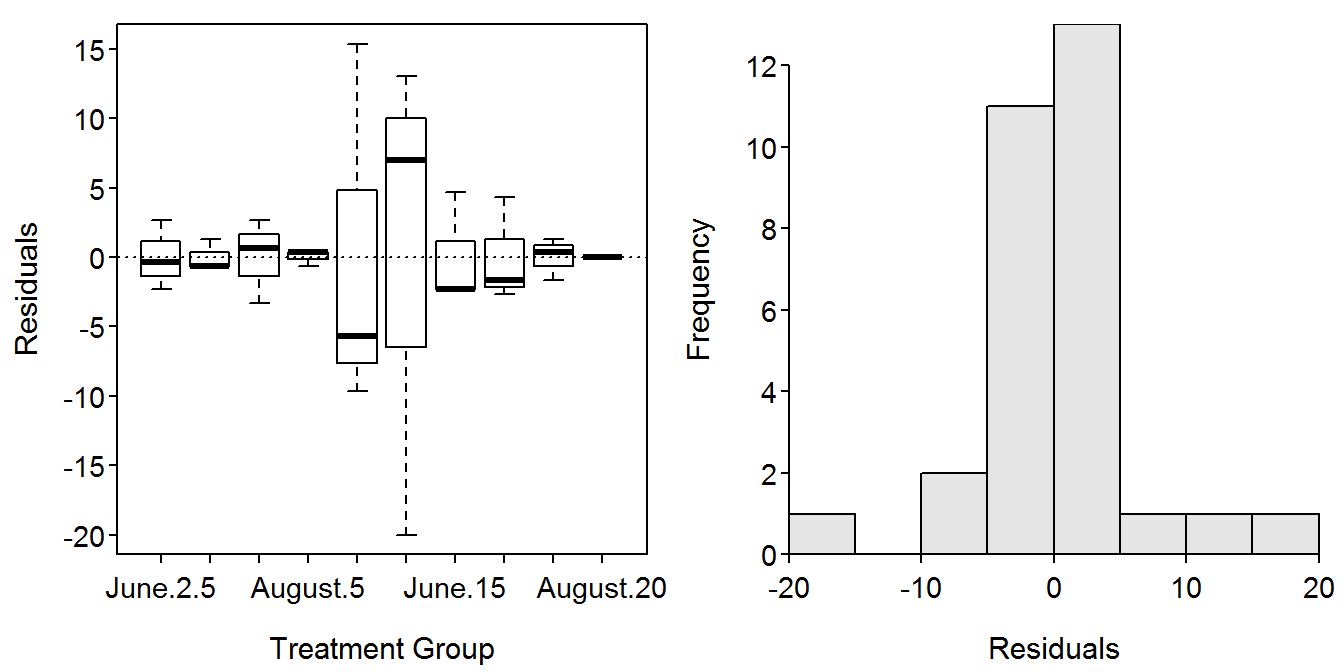> leveneTest(lm1)  Df F value Pr(>F) group 9 1.1233 0.3916 20  Finally, a histogram of the model residuals is constructed by including the residuals portion of the saved lm object into hist(). The results of the following command indicate that the combined residuals are approximately symmetric. > hist(lm1$residuals,main="")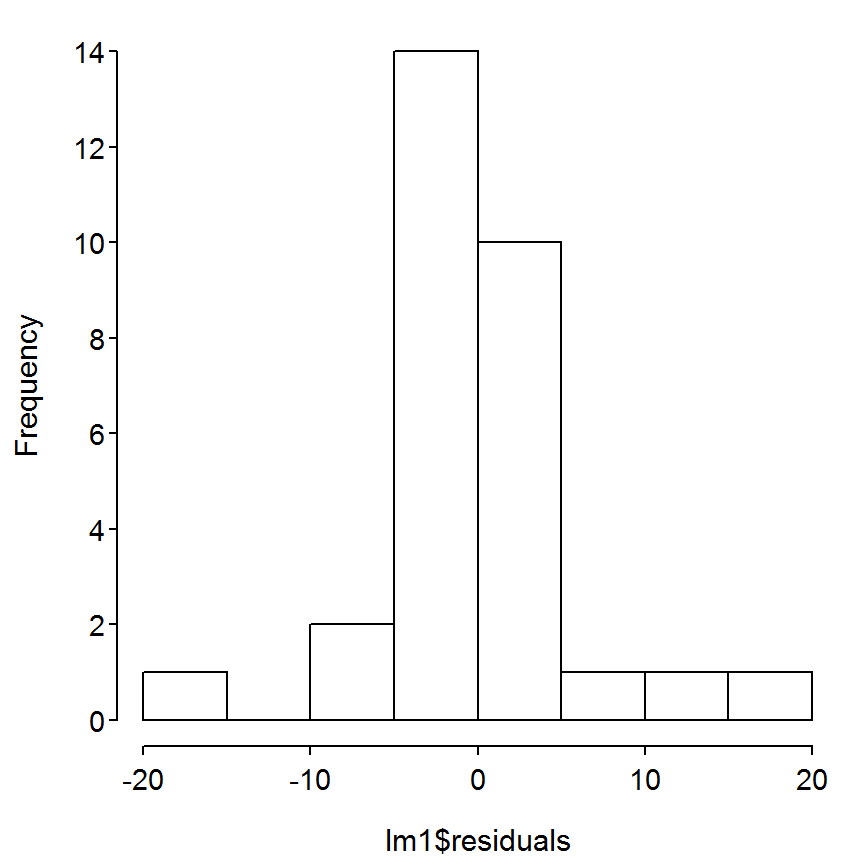## 7.6 Regression Analysis to Assess Habitat Features when $$\frac{C}{f}$$ Data are Used as the Response Variable

This hypothetical problem focuses on defining the habitat features affecting the densities of age-0 Smallmouth Bass (Micropterus dolomieu) around the shoreline of a natural lake in the Midwestern United States. The data is for 20 sites sampled along 50-m segments of shoreline of a Midwestern natural lake in late July. The mean bottom slope, proportion of the bottom composed of gravel-cobble substrate, and proportion of the bottom covered by aquatic macrophytes were measured at each site, and one pass was made at night with a boat-mounted electrofishing unit for age-0 Smallmouth Bass.

### 7.6.1 Preparing Data

The Box7_6.txt data file is read and observed below. In addition, cpe was transformed with common logarithms.

> d6 <- read.table("data/Box7_6.txt",header=TRUE)
> str(d6)
'data.frame':   20 obs. of  4 variables:
$cpe : int 0 5 1 10 12 0 1 3 25 0 ...$ gravel: num  0 5.2 0.3 5.5 6.7 0.8 0.1 1.9 8.3 0.5 ...
$veg : num 0 10.1 1.1 13.6 7.3 10.9 10 0 0 5 ...$ slope : num  7.3 1.5 2.2 1.2 1.7 5.3 8.3 3 1.5 4.3 ...
> d6$logcpe <- log10(d6$cpe+1)

### 7.6.2 Correlations

The authors examined the correlations between each pair of the three explanatory variables. This is accomplished by submitting a data frame of JUST the variables to cor(). The p-values, as shown in Box 7.6, are calculated with rcorr() from the Hmisc package. In contrast to cor(), rcorr() requires a matrix as the first argument. Fortunately, the first argument used in cor() can be included in as.matrix() and submitted as the first argument to rcorr().

> cor(d6[,c("gravel","veg","slope")])
            gravel         veg       slope
gravel  1.00000000  0.03203519 -0.64915855
veg     0.03203519  1.00000000 -0.09309214
slope  -0.64915855 -0.09309214  1.00000000
> rcorr(as.matrix(d6[,c("gravel","veg","slope")]))
       gravel   veg slope
gravel   1.00  0.03 -0.65
veg      0.03  1.00 -0.09
slope   -0.65 -0.09  1.00

n= 20

P
gravel veg    slope
gravel        0.8933 0.0020
veg    0.8933        0.6963
slope  0.0020 0.6963       

It is interesting to look at scatterplots corresponding to each pair of variables. The most efficient way to do this is to use pairs() with a “formula” as the first argument where each variable in the list is separated by a “plus sign” and the data frame in the data= argument. Note that the relationship between gravel and slope is clearly non-linear indicating that the value of the correlation coefficient is not strictly interpretable (i.e., the correlation coefficients assumes a linear relationship).

> pairs(~gravel+veg+slope,data=d6,pch=19)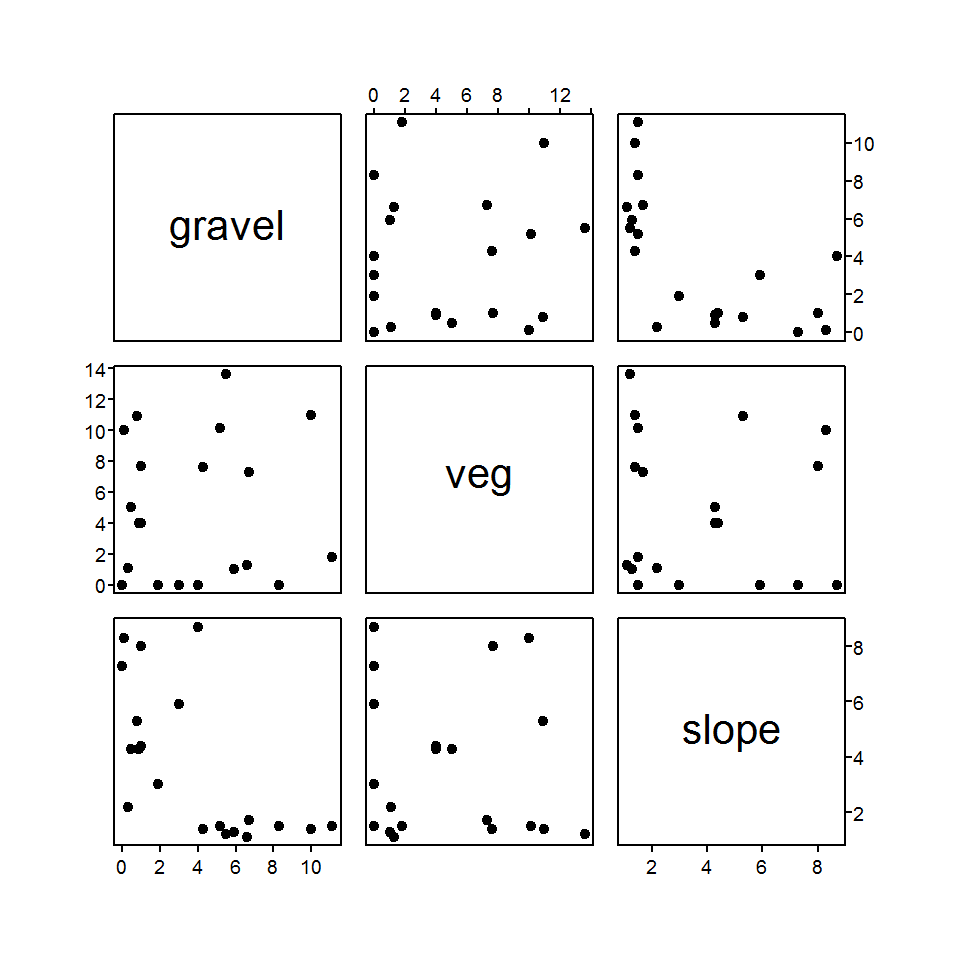### 7.6.3 Simple Linear Regressions

The authors constructed the regressions between cpe and all three explanatory variables and between logcpe and all three explanatory variables. Only the results from the regression of logcpe and gravel are shown. All regressions are computed with lm() as shown below.

> lm1 <- lm(cpe~gravel,data=d6)
> lm2 <- lm(cpe~veg,data=d6)
> lm3 <- lm(cpe~slope,data=d6)
> lm1a <- lm(logcpe~gravel,data=d6)
> lm2a <- lm(logcpe~veg,data=d6)
> lm3a <- lm(logcpe~slope,data=d6)
> anova(lm1a)
          Df Sum Sq Mean Sq F value   Pr(>F)
gravel     1 5.9315  5.9315  92.587 1.61e-08
Residuals 18 1.1532  0.0641
Total     19 7.0846                         
> summary(lm1a)
Coefficients:
Estimate Std. Error t value Pr(>|t|)
(Intercept)  0.04083    0.08608   0.474    0.641
gravel       0.16189    0.01682   9.622 1.61e-08

Residual standard error: 0.2531 on 18 degrees of freedom
Multiple R-squared: 0.8372, Adjusted R-squared: 0.8282
F-statistic: 92.59 on 1 and 18 DF,  p-value: 1.61e-08 
> confint(lm1a)
                 2.5 %    97.5 %
(Intercept) -0.1400153 0.2216778
gravel       0.1265402 0.1972332
> fitPlot(lm1a,xlab="Gravel (%)",ylab="log10(CPE+1)",main="")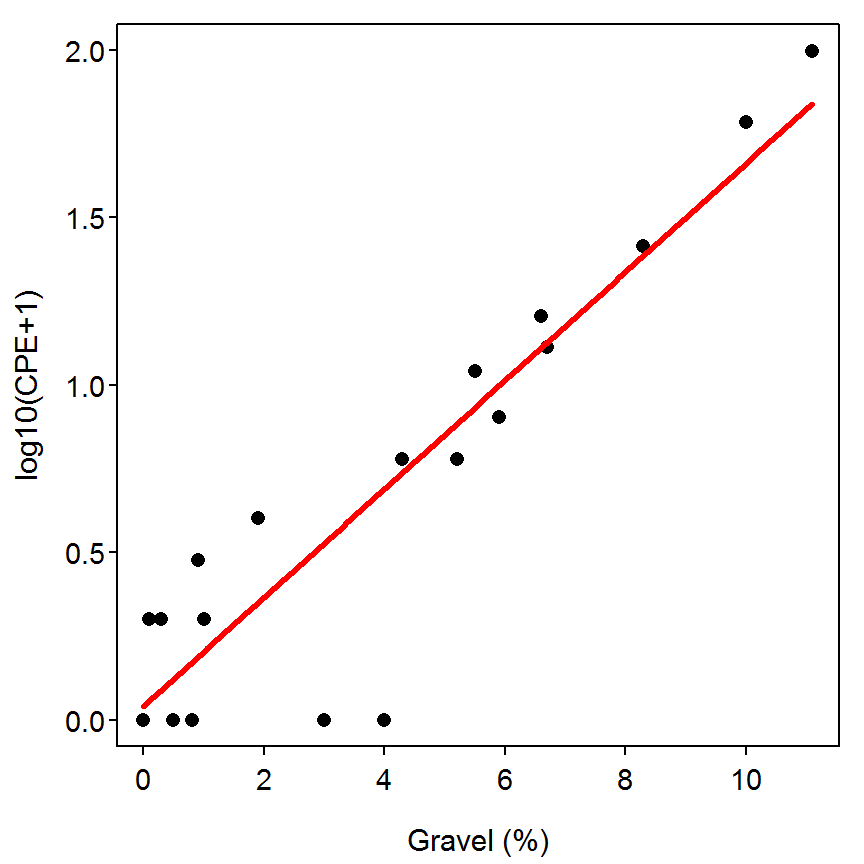### 7.6.4 Multiple Linear Regressions

The authors also fit a multiple linear regression model with the percentage of gravel, percentage of vegetation, and the interaction between the two as explanatory variables. The model is fit with lm() and the ANOVA table is extracted by submitting the saved lm object to anova(). As the authors note, neither the main effect of vegetation ($$p=0.3613$$) nor the interaction effect ($$p=0.9980$$) were significant. Thus, vegetation does not explain a significant portion of the variability in the log catch-per-unit-effort. Thus, it appears that gravel is the important variable in predicting log catch-per-unit-effort.

> lm4 <- lm(logcpe~gravel*veg,data=d6)
> anova(lm4)
           Df Sum Sq Mean Sq F value    Pr(>F)
gravel      1 5.9315  5.9315 86.8421 7.262e-08
veg         1 0.0603  0.0603  0.8831    0.3613
gravel:veg  1 0.0000  0.0000  0.0000    0.9980
Residuals  16 1.0928  0.0683
Total      19 7.0846                          

Reproducibility Information
Compiled Date: Wed May 13 2015
Compiled Time: 7:49:55 PM
Code Execution Time: 3.39 s

R Version: R version 3.2.0 (2015-04-16)
System: Windows, i386-w64-mingw32/i386 (32-bit)
Base Packages: base, datasets, graphics, grDevices, grid, methods, stats,
utils
Required Packages: FSA, car, Hmisc, multcomp and their dependencies
(acepack, cluster, codetools, dplyr, foreign, Formula, gdata, ggplot2,
gplots, graphics, grid, gridExtra, gtable, knitr, lattice,
latticeExtra, lmtest, MASS, methods, mgcv, mvtnorm, nnet, pbkrtest,
plotrix, proto, quantreg, relax, rpart, sandwich, scales, sciplot,
stats, survival, TH.data)
Other Packages: car_2.0-25, estimability_1.1, Formula_1.2-1, FSA_0.6.13,
lattice_0.20-31, lsmeans_2.17, multcomp_1.4-0, mvtnorm_1.0-2,
NCStats_0.4.3, nlstools_1.0-1, plotrix_3.5-11, rmarkdown_0.6.1,
survival_2.38-1, TH.data_1.0-6
boot_1.3-16, caTools_1.17.1, cluster_2.0.1, coda_0.17-1,
codetools_0.2-11, colorspace_1.2-6, DBI_0.3.1, digest_0.6.8,
dplyr_0.4.1, evaluate_0.7, foreign_0.8-63, formatR_1.2, gdata_2.16.1,
gplots_2.17.0, gridExtra_0.9.1, gtable_0.1.2, gtools_3.4.2, highr_0.5,
htmltools_0.2.6, KernSmooth_2.23-14, latticeExtra_0.6-26, lme4_1.1-7,
lmtest_0.9-33, magrittr_1.5, MASS_7.3-40, Matrix_1.2-0, mgcv_1.8-6,
minqa_1.2.4, munsell_0.4.2, nlme_3.1-120, nloptr_1.0.4, nnet_7.3-9,
parallel_3.2.0, pbkrtest_0.4-2, plyr_1.8.2, proto_0.3-10,
quantreg_5.11, RColorBrewer_1.1-2, Rcpp_0.11.6, relax_1.3.15,
reshape2_1.4.1, rpart_4.1-9, sandwich_2.3-3, scales_0.2.4,
sciplot_1.1-0, SparseM_1.6, splines_3.2.0, stringi_0.4-1,
stringr_1.0.0, tools_3.2.0, yaml_2.1.13, zoo_1.7-12